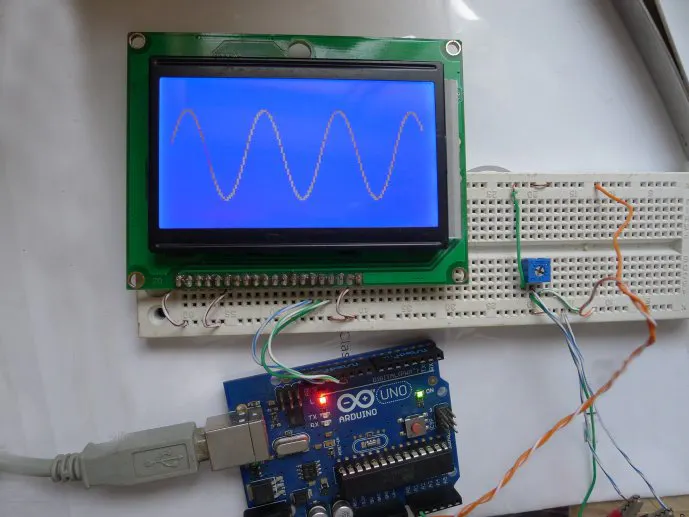Project tutorial# Digital Oscilloscope Experiment Based on Arduino

A digital oscilloscope experiment based on Arduino.

• 13,447 views
• 27 respects

## Components and supplies

Recently I used an Arduino for a digital oscilloscope experiment. It is very interesting to experiment with. The programming uses a u8g library file that supports multiple LCD displays. There is no need to know how a microcontroller works, nor care about the LCD driver. It makes programming very simple.

The display used is 12864 LCD driven by the ST7920 with an Arduino UNO. Here is the circuit diagram. (You can redeem it from PCBWay, please support me by registering with my invitation PCBWay link.)

### Step One

After running the basic code, the waveform of the input signal can be displayed.

Using the ADC inside the microcontroller for digital-to-analog conversion, up to about 1.5KHz input signal can be displayed.

Here is the basic code:

``````#include <U8glib.h>
U8GLIB_ST7920_128X64_4X u8g(13, 12, 11);
int x, y; int Buffer;
void setup( ) { }
void loop( )
{
for(x = 0;x < 128;x++)
u8g.firstPage();
do
{
for(x = 1;x < 127;x++)
u8g.drawLine(x, Buffer[x], x, Buffer[x+1]); }
while(u8g.nextPage( ));
}
``````

Waveform diagram as shown:

### Step Two: Add axes and borders

``````#include <U8glib.h>
U8GLIB_ST7920_128X64_4X u8g(13, 12, 11);
int x, y; int Buffer;
void setup( )
{
analogReference(INTERNAL);
}
void loop( )
{
for(x = 0;x < 128;x++)
u8g.firstPage();
do
{
for(x = 0;x < 127;x++)
u8g.drawLine(x, Buffer[x], x, Buffer[x+1]);
u8g.drawLine(64, 0, 64, 63);
u8g.drawLine(0, 32, 128, 32);
for(x=0;x<128;x+=8)
u8g.drawLine(x, 31, x, 33);
for(x=0;x<64;x+=8)
u8g.drawLine(63, x, 65, x);
u8g.drawFrame(0, 0, 128, 64);
}
while( u8g.nextPage( ));
}
``````

Here is the final version:

#### MQ-4 Natural Gas Sensor Experiment Based on Arduino

Project tutorial by Andrew85

• 13,687 views
• 12 respects

• 3,732 views
• 5 respects

• 54,211 views
• 132 respects

#### Arduino Based Digital Temperature Sensor

Project showcase by Abishek Bhalaaji

• 39,201 views
• 1 comment
• 21 respects

#### Arduino Oscilloscope

Project showcase by wayri

• 25,388 views